Varsity Math, Week 55________________

Whether or not it’s lucky, Varsity team member Sven likes the number seven, so he is playing with patterns created by seven points lying on the perimeter of a circle.

________________Diagonalization

The first thing that Sven does is draw the heptagon inside the circle whose vertices are the seven points. Then he starts to think about drawing in all the diagonals of that heptagon (line segments that join two non-adjacent vertices). It seems like it might take a long time…

How many diagonals does this heptagon have?

Regionalization

In the end Sven decides to draw all the diagonals, and he notices that between the heptagon itself and the diagonals, the original circle is broken up into many regions. (He also notices that for the seven points he’s chosen, there is no point through which three or more diagonals pass.) He wonders how many regions there are, and begins thinking about it by noticing that drawing all the lines between 2, 3, or 4 points on a circle splits the circle up into either 2, 4, or 8 regions (as shown in the diagram).Into how many regions do Sven’s heptagon and all its diagonals split the original circle?

Solutions to week 54

Watch for Falling Nuts. The second nut falls twice as far away from the tree as the second one, and it is critical to remember that the difference in distance means that it falls for twice as much time but it does not necessarily mean that it falls from twice the height. In fact, it has to fall from considerably higher than that in order to take twice the time to fall, since it is constantly speeding up due to the influence of gravity as it falls. In particular, the fact that the acceleration due to gravity is constant means that for any fall, the average speed of the nut is equal to the speed at the midpoint of the trip. So imagine that the first nut takes s seconds to fall, so that it has average speed 6/s meters per second. Then the second nut takes 2s seconds to fall, and its average speed is the same as its speed after s seconds, which has to the be the same as the first nut’s speed after s seconds, which is its final speed. But since the first nut is constantly accelerating, its final speed is twice its average speed. Hence, its final speed is 12/s meters per second. Therefore, the second nut’s average speed is 12/s meters per second, and it falls for 2s seconds, so it must have started from 24 meters up.

Fall Out of Line. This problem really led to a lot of creativity on the part of the Varsity Math team; there are lots of different possible ways to arrange the dominoes so that pushing A will make B fall but not the other way around. The conceptually simplest way is to simply rotate one of the middle dominoes 90 degrees so that it is lying on its long edge, and move it farther away from its neighbor toward A and closer to its neighbor toward B, so that when the dominoes are falling from A to B, the neigbor toward A will reach and tip over the sideways one, which is then close enough to tip over its neighbor toward B, but if it is tipped over from the other direction, its falling over will not reach the neighbor toward A. Another team member, wanting to know if it could be done with all of the dominoes vertical, made an extra-large gap and stacked dominoes three high vertically, so that falling from B to A can’t possibly reach A, but falling from A sends the top of the stack flying to knock over the dominoes toward B. A third team member, not satisfied with stacking dominoes, got them all standing in the ordinary way by creating a big chain reaction that would only be triggered in the falling direction from B which would knock away some intermediate tiles on the route from B to A before the chain could get there. And finally, a fourth member, concerned about the somewhat unpredictable dynamics of the “right-angle” knockout in the third solution, devised a way (using two forks of the type near B in that layout) so that when B is tipped over, the path to A is cancelled out by dominoes falling in the opposite direction, for the most reliable “one-way fall” of all. Photos of all four layouts, as actually tested by the Varsity Math team members, are below (the second two layouts are accompanied by pictures of what they look like after B is pushed over). In every photo, A is the leftmost domino and B the rightmost.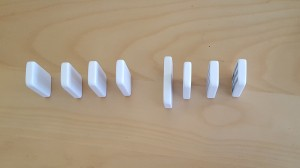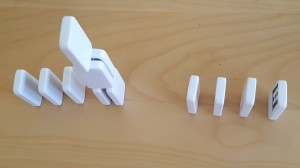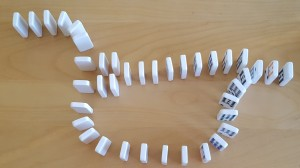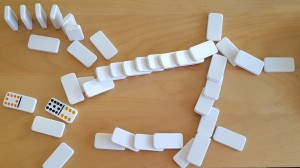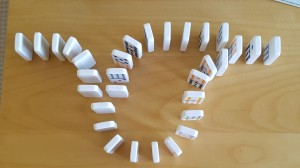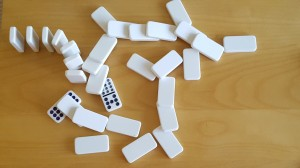Recent Weeks

Links to all of the puzzles and solutions are on the Complete Varsity Math page.

Come back next week for answers and more puzzles.

[asciimathsf]